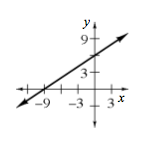### Home > CCA2 > Chapter 1 > Lesson 1.2.1 > Problem1-61

1-61.

Consider the equation $4x−6y=12$.

1. Predict what the graph of this equation looks like. Justify your answer.

Are there any exponents? What does this mean?

2. Solve the equation for $y$ and graph the equation.

Get $y$ by itself.

$-6y=-4x+12$

$\frac{-6y}{-6}=\frac{-4x+12}{-6}$

$y=\frac{2}{3}x-2$

3. Explain clearly how to find the $x$- and $y$-intercepts.

The $x$-intercept is the point where $y=0$.
The $y$-intercept is the point where $x=0$.

4. Which form of the equation is best for finding the $x$- and $y$-intercepts quickly? Why?

It’s easier to use the given, standard form of the equation to find the intercepts quickly because there is minimal rewriting involved.

5. Find the $x$- and $y$-intercepts of $2x−3y=−18$. Then use the intercepts to sketch a graph quickly.

Set $x=0$ to find the $y$-intercept, and $y=0$ to find the $x$-intercept.

$2(0)−3y=−18$

$x$-intercept: $(−9,0)$
$y$-intercept: $(0,6)$